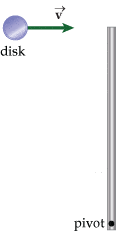# Angular Momentum; rod & disk inelastic collision

## Homework Statement

The figure shows an overhead view of a 2.50-kg plastic rod of length 1.20 m on a table. One end of the rod is attached to the table, and the rod is free to pivot about this point without friction. A disk of mass 39.0 g slides toward the opposite end of the rod with an initial velocity of 33.0 m/s. The disk strikes the rod and sticks to it. After the collision, the rod rotates about the pivot point.(a) What is the angular velocity of the two after the collision?

What is the angular momentum of an object moving with a linear velocity, about a given point? rad/s

(b) What is the kinetic energy before and after the collision?
KEi
= J
KEf
= J

## Homework Equations

L=Iw
(Iw+Iw)I=(iw+iw)f
w=v/r
KErot=1/2Iw^2
Irod=ML^2/3
Idisk=MR^2/2

## The Attempt at a Solution

I believe the set up would look something like this:
(Irodxwrod)i+(Idisk x wdisk)I=(Idisk+Irod)wf
The initial speed of the rod is zero, canceling that out.
MR^2/2 x V/R=(MR^2/2 + ML^2/3)wf
The problem I am having is we are not given the radius of the disk. Is it possible I am missing that the radius cancels out?

And again, with the KE, we need the radius in order to solve for angular momentum in the equation.

Thank you for any help!

kuruman
Homework Helper
Gold Member
Assume that the disk is a point mass. It appears you are conserving angular momentum. About what point is angular momentum conserved?

Ok, so if this is a point mass I=MR^2, where r would be the distance from mass to the axis. So in this case, 1.2? Would this be applied on both the initial and final sides of the equation? I can see how it would be a point mass when attached to the pole, but why when it is solo?

And to answer what point angular momentum conserved, would this be at the pivot point?

I apologize but I do not know what LaTex is!

Thank you so much for your help.

kuruman
Homework Helper
Gold Member
Ok, so if this is a point mass I=MR^2, where r would be the distance from mass to the axis. So in this case, 1.2?
Yes, but call the length of the rod L and put in numbers at the very end. Also, the mass of the disk is not equal to the mass of the rod, so use different symbols for the two.
I can see how it would be a point mass when attached to the pole, but why when it is solo?
Because, as you correctly pointed out, you are not given the radius of the disk, therefore you are to assume it's a point mass. If it were an extended mass, you would have to worry about exactly where on the rod its center of mass is stuck.
And to answer what point angular momentum conserved, would this be at the pivot point?
Yes. That's also the point about which you calculate all moments of inertia.
apologize but I do not know what LaTex is!
Look all the way to the left of the POST REPLY button. Click LaTeX to the right of the question mark. It is a powerful, easy-to-learn equation writer that you should invest some time in.

So here is my final set up.

MdiskR^2 x V/R=(MdiskR^2 + MrodL^2)w
which can simplify to
Mdisk xR x V=(Mdisk R^2 + Mrod L^2) w

I tried to make it clear-I will check out the LaTex when I have a little more time.

would this be an accurate set up? I obtained .4212 rad/sec, which seems small.

kuruman
Homework Helper
Gold Member
What is the moment of inertia of a rod about its end?

ah I forgot! ML^2/3

Mdisk R^2 x V/R=(MdiskR^2 +Mrod L^2/3) w

I now obtained 1.226 rad/sec, which seems more acceptable.

kuruman
Homework Helper
Gold Member
I trust you used R = L when you put in the numbers.

Yes! And this was the correct answer. THANK YOU for your help!

And one more question if you don't mind. For the KE after the collision, I understand this is now rotational kinetic energy which is 1/2Iw^2. We would use the w found in part 1, but for the moment of inertia would it be the combined values for the disk and rod? So:

KEf=1/2 (Mdisk R^2 + [Mrod L^2/3]) w^2

kuruman